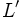# Derived subring of a Lie ring

Jump to: navigation, search

## Definition

Let$L$ be a Lie ring. The derived subring or commutator subring of$L$, denoted$L'$, or$[L,L]$ is defined in the following ways:

• It is the additive subgroup generated by all elements of the form$[x,y]$, where$x,y \in L$
• It is the Lie subring generated by all elements of the form$[x,y]$, where$x,y \in L$
• It is the Lie ideal generated by all elements of the form$[x,y]$, where$x,y \in L$

In situations where the Lie ring is an algebra over some field or ring, the derived subring is also a subalgebra and an ideal over that field or ring. In those cases, it may be termed the derived subalgebra or commutator subalgebra.